• No products in the cart.

# 301.4.4-Functions

### Functions

In this section we will talk about the functions in the pig. Function is very important concepts while doing the analysis we might use several functions like “sum, count, average, summary functions, numerical functions , string functions and etc.”” Writing the map reduce code for each one them is really a tiring task But in the pig there are some generic functions which automatically gets converted in to the map reduce code and make the analysis more interesting. Lets start learning the functions .

#### Count function

Lets start learning the functions . First function is the count , which we had already saw in the previous lectures. For every functions we have to start by “GROUP by” keyword then apply the respective function for each row. First we will start with “Count function” For this example we will be using the relation “Retail_Customer_pig

• We need to group the record first
• We can use FOREACH and GENERATE operator to generate counts
``````group_country = GROUP Retail_Customer_pig by Country;
Counts_country= FOREACH group_country GENERATE group, COUNT(Retail_Customer_pig);``````

Here we trying to count the number of the customer for each country the way we are trying to do is, for each group country generate group name that is country name and the count which will be store in the new relation called as “Counts_country”.

Next we can dump the “counts_country” relation which will give us the country name and the number of customers associated with those country.

``    DUMP Counts_country;``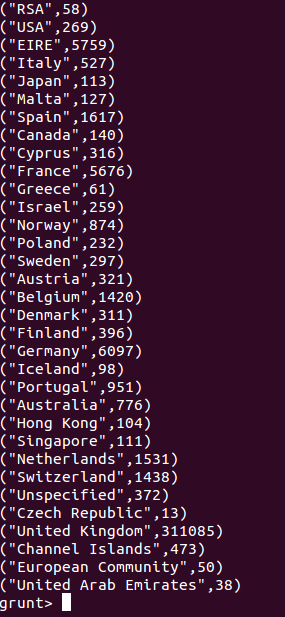By viewing the output we can say that country “RSA have 58 customers” country “USA have 269 customers” and so on.

#### Sum function

Next we will work on the “SUM” function lets us see how dose the sum function works.

First step is again we need to do the group by, in this particular example we will do group by country . Next snippet of the code will do the sum of the unit price by the country names , lets try to understand this code in more detail SUM_COUNTRY which is the new relation name.

``````    group_country = GROUP Retail_Customer_pig by Country;
SUM_country= FOREACH group_country GENERATE group, SUM(Retail_Customer_pig.UnitPrice);
``````

FOREACH group_country means , we know that “group_country” is a relation which is a bag consist of country name and all the rows associated with it. “GENERATE group”” that is country name SUM(Retail_Customer_pig.UnitPrice); this will do the sum of the unit prices. Basically the output we are looking for country name and the sum of the unit price.

Lets print the output and see are we getting the desired output or not . Printing of the output can be done by DUMP command

``    DUMP SUM_country;``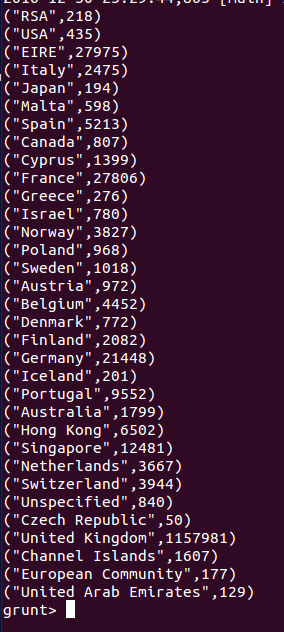So we got the output in the format of “country name and sum of the unit price”.

#### AVG function

Lets find the average unit price by each country. As usual we have to do group by in the first step, for this example we are doing group by country . Once we have the country values we can continue further. So for each country in that group country relation generate group that is nothing but the country name and the average of the unit price from this operation.

• Average by country
``````group_country = GROUP Retail_Customer_pig by Country;
AVG_country= FOREACH group_country GENERATE group, AVG(Retail_Customer_pig.UnitPrice);``````

Now we will dump it and check the results .

``````        DUMP AVG_country;

``````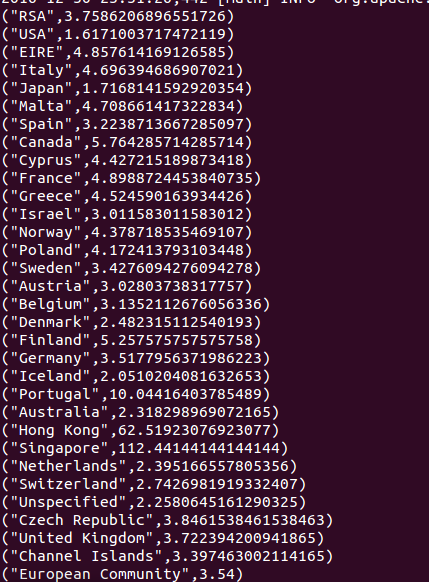The results are printed in the format of the country name and the average unit price.

### Arithmetic Functions on Overall Data

Now the question arise is that if we wants to find the average of the overall dataset or the average of the whole dataset then how can we do it. Or the question can be frame in this way, we don’t want the average by each country we want only the average of the unit price for this whole datasets. Here there is a problem all these functions works only on “group by” only so first we have to do the group by then only we can use for each followed by the function . Without preforming the group by operation we cant perform this task. So here we can do the “group by all” Earlier we were trying to do the group by the country but here “group by all” will group everything and keep it in one bag.

• What if we want the overall sum and overall average? Not group-wise summary.
• We can use GROUP ALL
• Group all will give the same dataset as result. Then we can use FOREACH and GENERATE

#### Overall Average

``````    group_all = GROUP Retail_Customer_pig ALL;
All_avg= FOREACH group_all GENERATE  AVG(Retail_Customer_pig.UnitPrice);
DUMP All_avg;``````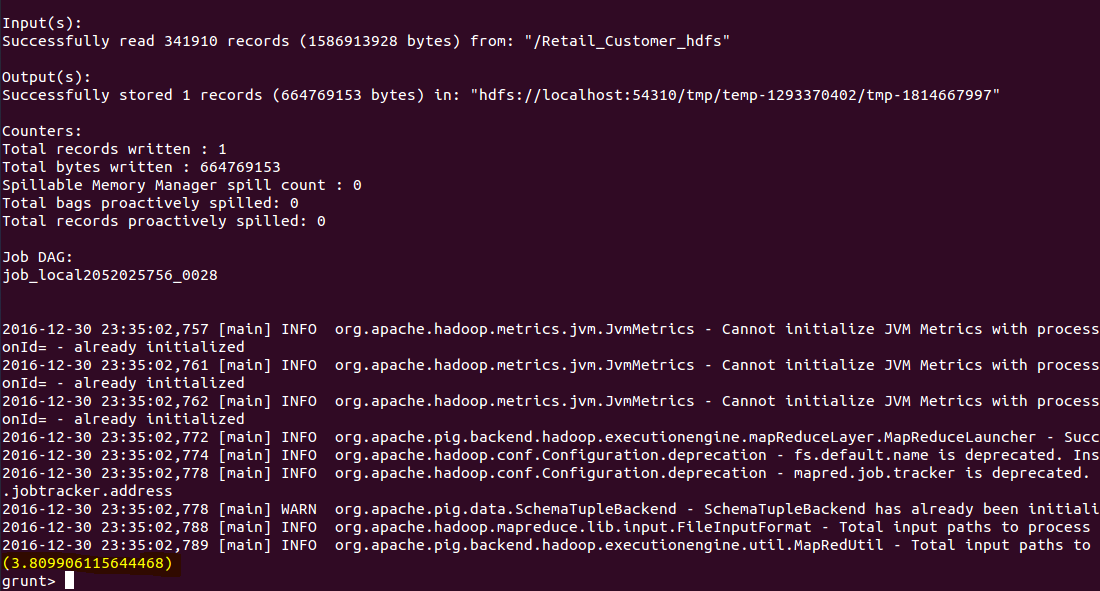#### Overall Sum and Count

Usually we have to use group by all, where a single group is created by using all the observations code “group_all = GROUP Retail_Customer_pig ALL;” After this we can do all sum the code is similar to above codes so detailed explanation is not necessary here. “All_sum= FOREACH group_all GENERATE SUM(Retail_Customer_pig.UnitPrice);” Then we can do all count where the overall count of the unit price will be generated and stored in the relation called “All_Count” Then we can print sum by using the dump command . And we print count by using same dump command.

``````group_all = GROUP Retail_Customer_pig ALL;
All_sum= FOREACH group_all GENERATE  SUM(Retail_Customer_pig.UnitPrice);
All_Count= FOREACH group_all GENERATE  COUNT(Retail_Customer_pig.UnitPrice);
DUMP All_sum;
DUMP All_Count;``````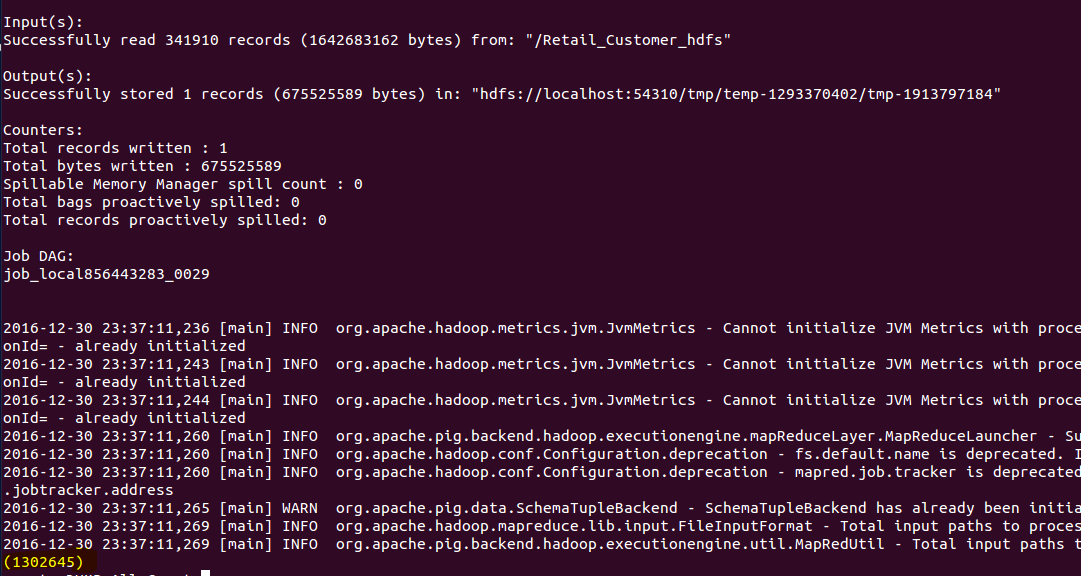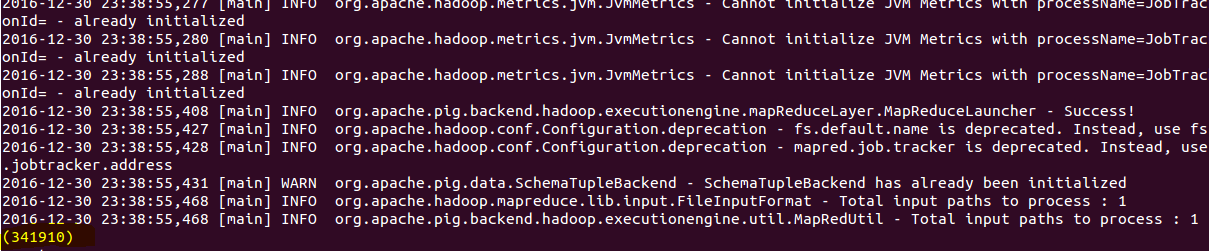• ABS
• ACOS
• ASIN
• ATAN
• CBRT
• CEIL
• COS
• COSH
• EXP
• FLOOR
• LOG
• LOG10
• RANDOM
• ROUND
• SIN
• SINH
• SQRT
• TAN
• TANH

### Substring Function

• SUBSTRING(string, startIndex, stopIndex) – for each record
``````DESCRIBE  Retail_invoice_pig1;
Invoice_Substr= FOREACH Retail_invoice_pig1 GENERATE InvoiceNo, SUBSTRING(StockCode, 2,6) as code_sub , Quantity;
DESCRIBE Invoice_Substr;
DUMP Invoice_Substr;``````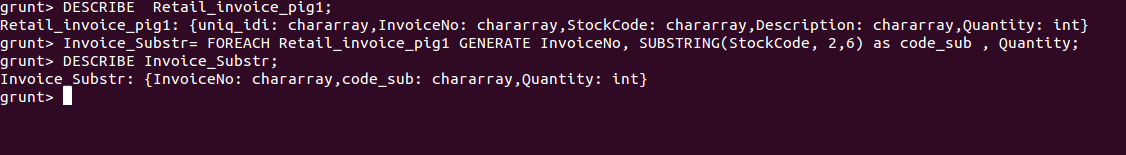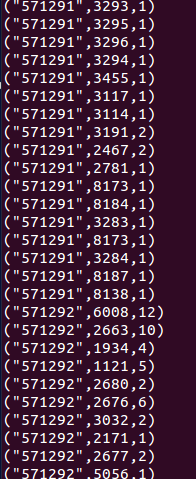### Other String Functions

• INDEXOF
• LAST_INDEX_OF
• LCFIRST
• LOWER
• REGEX_EXTRACT
• REGEX_EXTRACT_ALL
• REPLACE
• STRSPLIT
• SUBSTRING
• TRIM
• UCFIRST
• UPPER

22nd March 2018
0 responses on "301.4.4-Functions"